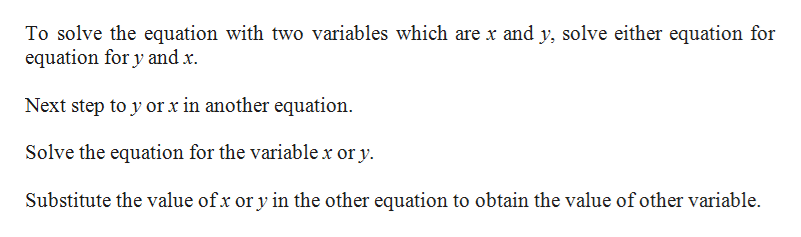# Two algebraic methods to solve a system of linear equations in two variables are the __________ method and the __________ method.

Question
1 views

Two algebraic methods to solve a system of linear equations in two variables are the __________ method and the __________ method.

check_circle

Step 1

Find the two algebraic methods to solve the equations with two variables.

Step 2

The two methods are substitution me...help_outlineImage TranscriptioncloseTo solve the equation with two variables which are x and y, solve either equation for equation for y and x. Next step to y or x in another equation. Solve the equation for the variable x or y. Substitute the value of x or y in the other equation to obtain the value of other variable. fullscreen

### Want to see the full answer?

See Solution

#### Want to see this answer and more?

Solutions are written by subject experts who are available 24/7. Questions are typically answered within 1 hour.*

See Solution
*Response times may vary by subject and question.
Tagged in

### Other